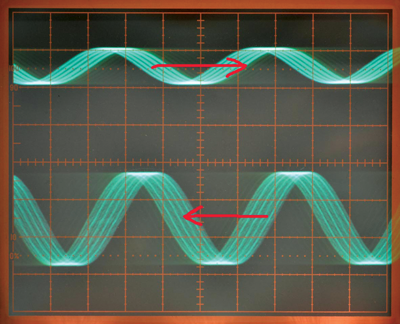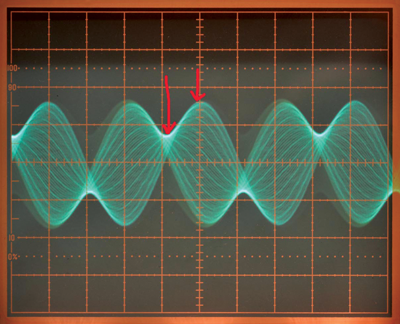# A Method of Demonstrating Transmission-Line Behavior on a Dual-Channel Oscilloscope

### 要約

This application note describes a novel way to visualize standing waves on a transmission line, using a dual-channel oscilloscope. This oscilloscope displays two sine waves of precise frequency generated via direct digital synthesis (DDS), using a Maxim® MAX5715 digital-to-analog converter (DAC), which is interfaced to an Atmel® AVR processor.

A similar version of this article was published December 22, 2013 in EDN.

### Introduction

In the past, demonstrations of standing-wave phenomena could be done using an actual, physical transmission-line setup called “Lecher Lines.”1 This method required laborious measurements to determine the standing wave pattern. The measurements themselves, moreover, could disturb the pattern, and there was certainly no instantaneous visual representation of the pattern.

There is a novel way to visualize standing waves on a transmission line using a dual-channel oscilloscope. A well-known characteristic of oscilloscopes is that when the sweep is not synchronized to the displayed waveform but is close to its frequency or a submultiple, then the waveform appears to move on screen. It will move to the right if the sweep is running above the waveform frequency or a submultiple, and to the left if the sweep is below the waveform frequency or a submultiple. If a waveform of a frequency below the sweep frequency is applied to one channel of a dual-channel oscilloscope, and a waveform of a frequency above the sweep frequency is applied to the other channel, the two waves will appear to move in opposite directions. They could, in fact, represent the forward and reflected waves on a transmission line. Further, switching the oscilloscope to Add mode displays a single waveform that represents the resulting standing wave.

Creating a meaningful display requires sine waves of precise frequency which is easily done using direct digital synthesis (DDS). This demonstration uses frequencies around 400Hz because this provides a visually appealing and easily photographed display. Three waveforms are generated: 399Hz and 401Hz sine waves, and a 400Hz stepped wave for synching (triggering) the oscilloscope. The 1Hz difference between each wave and the scope sweep triggering frequency gives an apparent 1Hz signal frequency on the simulated transmission line. Although the scope trigger frequency is 400Hz, the sweep speed is set to run at a submultiple of 400Hz so that several cycles of each wave are visible.

### The Display

Figure 1 shows how the two sine waves appear on screen in dual-trace mode.The arrows show the direction of motion on screen. The effect is hard to see in a still picture, so a video is also available

The demo presented here runs best on an old-fashioned analog oscilloscope. The display on a digital scope may be too “jumpy,” depending on its update rate. There is, nonetheless, one way in which a digital scope is superior to an analog scope for the demo, and it is described below.

Figure 2 shows the resultant standing wave when the oscilloscope is switched to Add mode.Again, it is hard to see the effect in a still picture. Here five nodes (points which are always at zero voltage) can be seen. Between them, the wave oscillates from its negative (limit) to its positive limit. In this case, the forward and reflected waves are equal, so the transmission line must be either open or shorted. If the termination is at the left, it must be a short since a node is located there. The VSWR is the ratio of maximum to minimum amplitudes of the standing wave. Since the minimum amplitude is zero, the VSWR is infinite.

Other termination conditions can be simulated by varying the gain of one of the oscilloscope channels. In Figure 3, the return amplitude (upper trace) is reduced by half. This corresponds to a termination that is neither open nor short, nor the characteristic impedance of the line.The resulting standing wave pattern is seen in Figure 4.There are no nodes (points of zero voltage). Instead, the amplitudes of the waveform at the maximum and minimum, as indicated by the arrows, show a ratio of 2 to 1, simulating a VSWR of 2:1.

### Direct Digital Synthesis

The generation of exact frequencies is necessary for a stable display. Here “stable” means that the horizontal positions of the nodes, or the maxima or minima of the standing wave pattern, do not move on the display. An implementation of DDS using three numerically controlled oscillators (NCOs)2 is used. The NCOs are implemented with 16-bit counters called phase accumulators that are incremented at a fixed clock frequency, FCLOCK, by an amount called the tuning word, or frequency control word, FCW (Figure 5). The FCLOCK is much higher than the waveform frequency.The eight most significant bits of the phase accumulator are applied to a sine lookup table (LUT), and the table value is sent to the DAC. The value in the phase accumulator can be thought of as defining a point on a circle; the tuning word defines the “stride length” of a walk around the circle. The larger the tuning word, the faster the trip around the circle and, thus, the higher the frequency of the output. The resulting frequency is given by this expression:

FOUT = FCW × FCLOCK/216

The output frequency is directly proportional to FCW. In this case, FCLOCK is 31.25kHz. FCW values of 841, 839, and 837 are used, giving these frequencies:

F1 = 841 × 31250/65536 = 401.02Hz

F2 = 839 × 31250/65536 = 400.06Hz

F3 = 837 × 31250/65536 = 399.11Hz

The frequencies are expressed to two decimals here, but actually (F1 - F2) is exactly equal to (F2 - F3). The difference, to nine decimal places, is 0.953674316Hz.

### The Implementation

This demo is implemented using three channels of a MAX5715 4-channel 12-bit digital-to-analog converter (DAC) driven by an Atmel® AVR processor on an Arduino® Uno board. The Arduino is an open-source hardware and software platform, convenient as a development environment for AVR-based systems. The Maxim DAC is easily interfaced to the Arduino using SPI (serial peripheral interface), which is supported by the AVR hardware and by a library provided as part of the Arduino software. Routines from the Arduino SPI library are used to initialize the AVR SPI hardware. Routines are also available to do the actual SPI data transfer, but these were too slow and were not used.

The three phase accumulators are 16-bit counters, which are incremented in an interrupt routine driven by one of the processor’s timers, causing an interrupt every 32µs (1/31.25kHz). Two of the phase accumulator values (generating F1 and F3) are converted to sines with a LUT and sent to two channels of the DAC. The two most significant bits of a third phase accumulator (generating F2) are sent to the third DAC channel to generate a 4-step scope trigger signal. By varying the trigger level, the displayed phase of the standing wave pattern can be chosen to simulate an open or shorted termination.

The Arduino SPI library data-transfer routines were so slow that they extended the interrupt routine duration beyond the interrupt period. Consequently, code to directly write to the AVR SPI hardware was used instead.

The interrupt routine code:

```//******************************************************************
// Timer2 Interrupt Service at 31372.550 Hz = 32uSec
// this is the timebase REFCLOCK for the DDS generator
// FOUT = (M (REFCLK)) / (2 exp 32)

ISR(TIMER2_OVF_vect) {

// set the SS line low
PORTB &= (0xFF ^ 4);
//  send in the address and value via SPI:
SPDR = CODEA;
// update phase accumulators while waiting
phaccu_a += tword_a;
phaccu_b += tword_b;
phaccu_c += tword_c;
while (!(SPSR & _BV(SPIF)))
;
// do the table lookup based on accum 'a' high-order byte
while (!(SPSR & _BV(SPIF)))
;

SPDR = 0;
while (!(SPSR & _BV(SPIF)))
;
// set the SS line high
PORTB |= 4;
// set the sS line low for the second SPI transfer
PORTB &= (0xFF ^ 4);
// do the second SPI transfer
SPDR = CODEB;
while (!(SPSR & _BV(SPIF)))
;
// do table lookup based on accum 'b'
while (!(SPSR & _BV(SPIF)))
;
SPDR = 0;
while (!(SPSR & _BV(SPIF)))
;
// take the SS pin high to de-select the chip:
PORTB |= 4;

// set the sS line low for the third SPI transfer
PORTB &= (0xFF ^ 4);
while (!(SPSR & _BV(SPIF)))
;
// Four stairsteps on output C
SPDR = highByte(phaccu_c) & 0xC0;
while (!(SPSR & _BV(SPIF)))
;
SPDR = 0;
while (!(SPSR & _BV(SPIF)))
;

// take the SS pin high to de-select the chip:
PORTB |= 4;

}
```

The connections between the Arduino and the MAX5715 are shown in Figure 6.The MAX5715 was soldered to an adapter board and then to an Arduino Uno prototype “shield” (Figure 7). (“Shields” are boards that provide various add-on functions to the Arduino system.)### The Display on a Digital Oscilloscope

As mentioned above, this demo works best on an analog oscilloscope, because the display on a digital oscilloscope may be jumpy depending upon the update rate. But a digital oscilloscope’s ability to simulate a long-persistence display shows the standing wave pattern well, as in Figure 8.### Conclusion

The usual goal in using an oscilloscope is a nonmoving display. This application note used a controlled moving display to show what really happens on a transmission line.